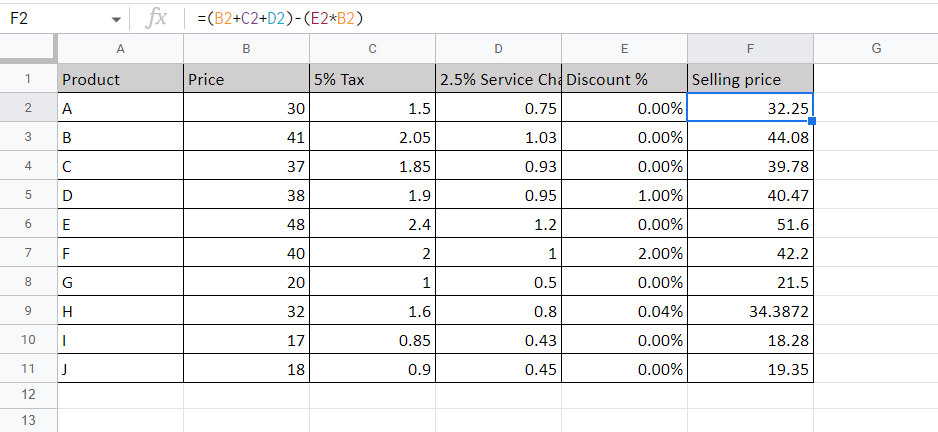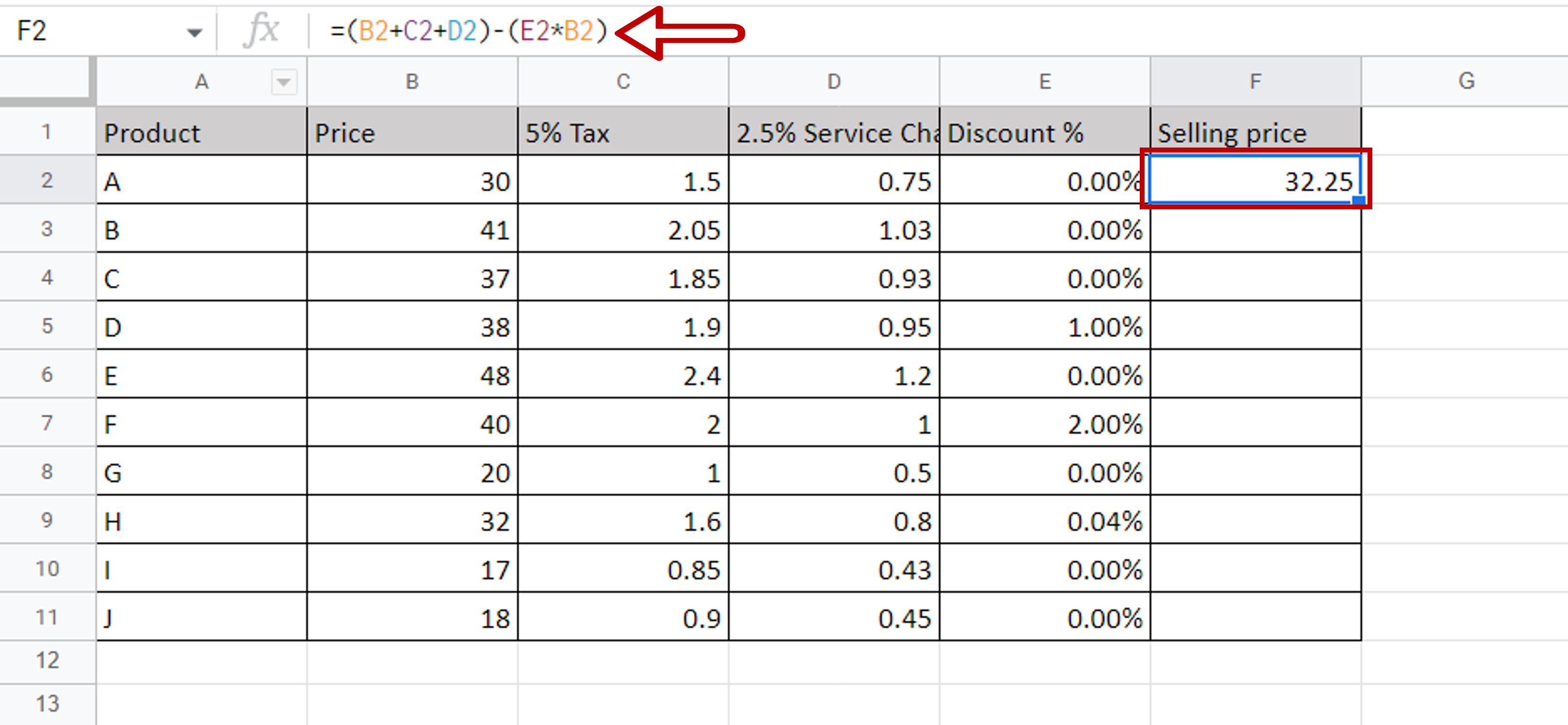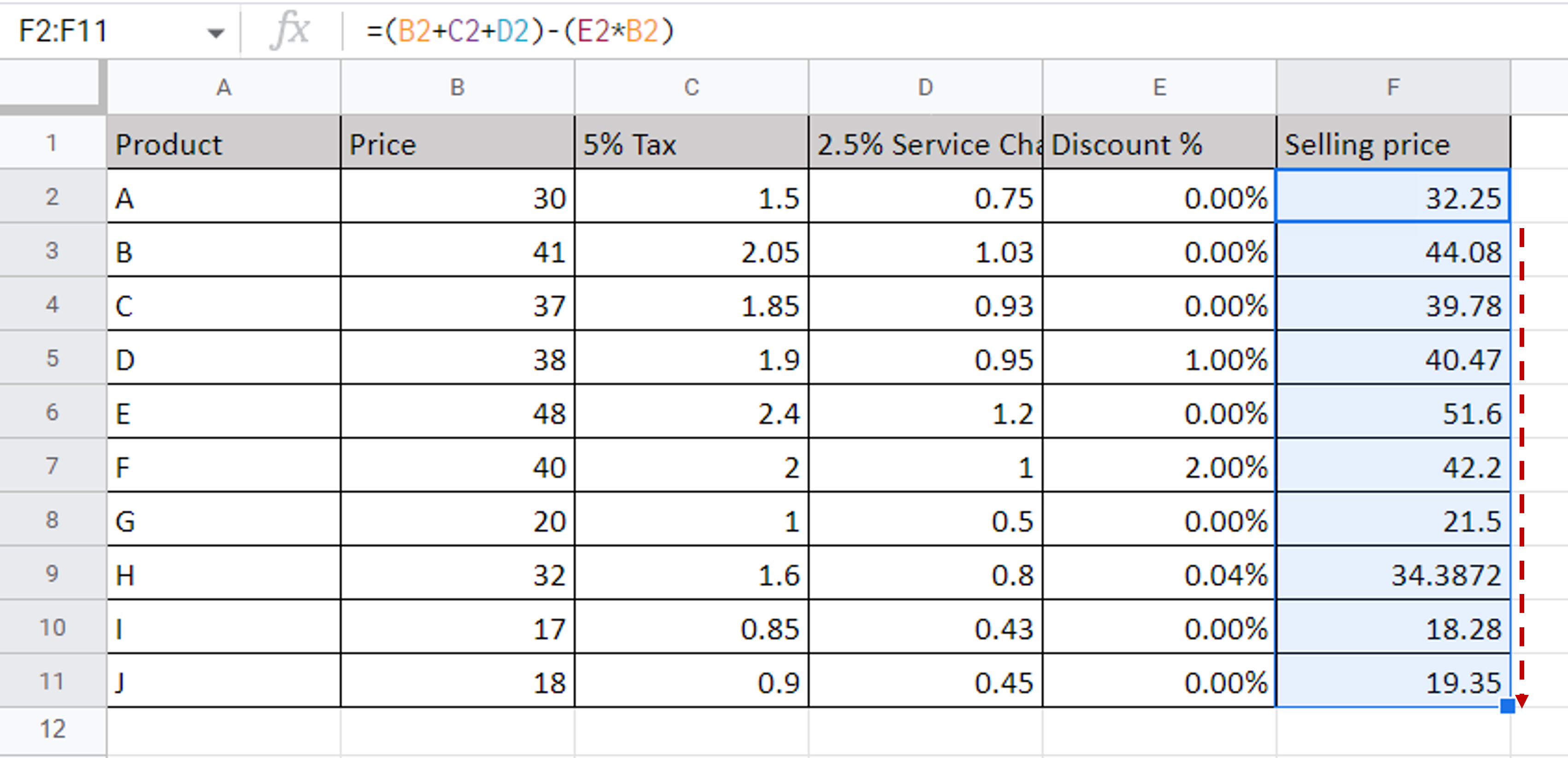# How to do equations in Google Sheets

You can watch a video tutorial here.One of the great things about Google Sheets is the ease with which complex formulas and calculations can be done. Using mathematical operators equations can be built and then copied to be applied across rows or columns.

In this example, we create an equation to compute the selling price:

Selling price = (Price + 5% Tax + 2.5% Service Charge) – (Discount % * Price)

We add tax and service charges to the product price, remove the discount, if any, and arrive at the final selling price.

### Step 1 – Type the equation– In the destination cell type the formula using the cell references:
=(Price + 5% Tax + 2.5% Service Charge) – (Discount % * Price)

### Step 2 – Copy the formula– Using the fill handle from the first cell, drag the formula to the remaining cells
OR
a) Select the cell with the formula and press Ctrl+C or choose Copy from the context menu (right-click)
b) Select the rest of the cells in the column and press Ctrl+V or choose Paste from the context menu (right-click)